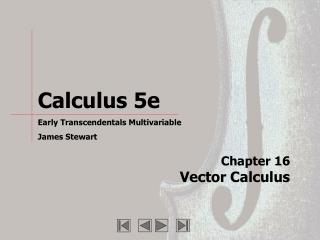# Chapter 16 Vector Calculus - PowerPoint PPT PresentationDownload PresentationChapter 16 Vector Calculus

Chapter 16 Vector CalculusDownload Presentation## Chapter 16 Vector Calculus

- - - - - - - - - - - - - - - - - - - - - - - - - - - E N D - - - - - - - - - - - - - - - - - - - - - - - - - - -
##### Presentation Transcript

1. Chapter 16Vector Calculus

2. Animations Follow the link to the slide. Then click on the figure to play the animation. A • Figure 16.3.9 • Figure 16.6.5 • Figure 16.6.10

3. Section 16.1 · Figure 1 Air velocity vectors indicating wind speed and direction (b) 4:00 P.M., June 30, 2002 (a) 12:00 P.M., June 11, 2002

4. Section 16.1 · Figures 10-12 Examples of computer-generated vector fields

5. Section 16.1 · Exercises 11-14 I II 11. 12. 13. 14. IV III

6. Section 16.1 · Exercises 15-18 15. 16. 17. 18. I II IV III

7. Section 16.3 · Figure 9 The vector field in Example 3 A

8. Section 16.6 · Figure 5 Grid curves for A Animate v constant Animate u constant A

9. Section 16.6 · Figure 10 Computer-generated plot of the graph in Example 8 A

10. Section 16.6 · Exercises 11-16 III I II IV V VI

11. Section 16.10 · Summary Box × × × Summary: Higher-dimensional versions of the Fundamental Theorem Fundamental Theorem of Calculus Fundamental Theoremfor Line Integrals Green’s Theorem Stokes’ Theorem Divergence Theorem ×